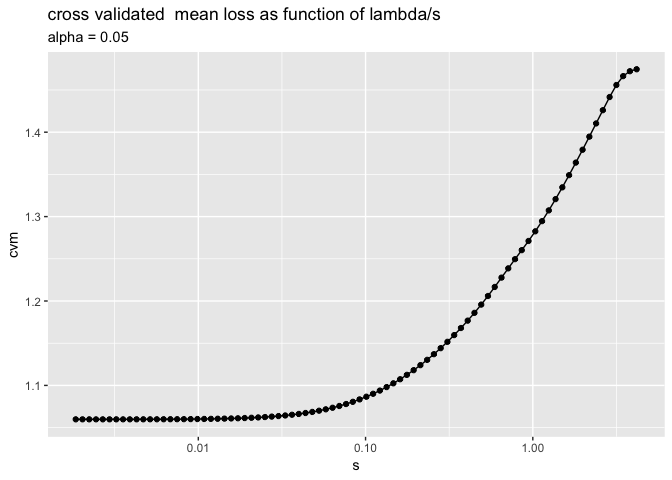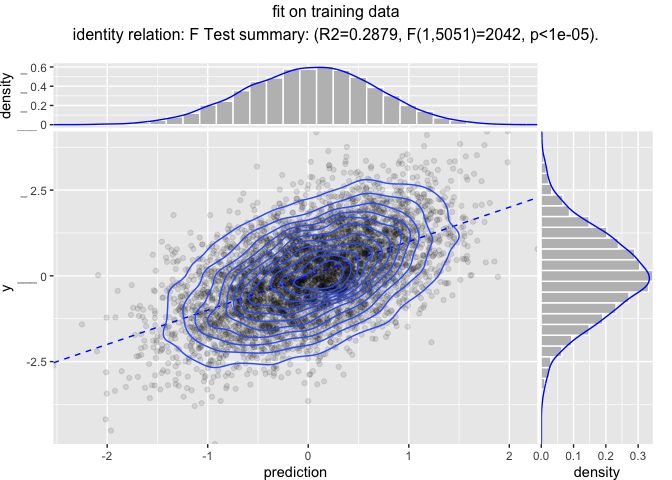# Sharing Modeling Pipelines in R

Reusable modeling pipelines are a practical idea that gets re-developed many times in many contexts. `wrapr` supplies a particularly powerful pipeline notation, and a pipe-stage re-use system (notes here). We will demonstrate this with the `vtreat` data preparation system.

Our example task is to fit a model on some arbitrary data. Our model will try to predict `y` as a function of the other columns.

Our example data is 10,000 rows of 210 variables. Ten of the variables are related to the outcome to predict (`y`), and 200 of them are irrelevant pure noise. Since this is a synthetic example we know which is which (and deliberately encode this information in the column names).

The data looks like the following:

``str(d)``
``````## 'data.frame':    10000 obs. of  211 variables:
##  \$ y        : num  -1.086 -1.246 -0.173 -1.087 1.661 ...
##  \$ var_001  : num  0.0108 NA -0.0149 -0.2192 -0.1605 ...
##  \$ var_002  : num  -3043966 NA -2151778 -4942298 455479 ...
##  \$ var_003  : num  -60627 -34317 64535 -1940 NA ...
##  \$ var_004  : num  -373812 NA -232051 21812 74992 ...
##  \$ var_005  : num  29370 -296583 -57431 -52728 94272 ...
##  \$ var_006  : num  -108112 NA -387325 -803572 -60534 ...
##  \$ var_007  : num  19294 -85200 498536 -52584 -14377 ...
##  \$ var_008  : num  -253 -1296 -163 -568 -862 ...
##  \$ var_009  : num  -2.13e+09 3.96e+10 -4.20e+10 -1.11e+10 -6.93e+10 ...
##  \$ var_010  : num  148.15 997.9 5.92 -552.88 NA ...
##  \$ noise_001: num  1.61e-07 -5.21e-07 NA -8.80e-08 9.76e-07 ...
##  \$ noise_002: num  854212 -221108 -654245 -1095501 NA ...
##  \$ noise_003: num  -53443 -28367 NA 8445 21881 ...
##  \$ noise_004: num  3422424 NA 2538108 -2222437 NA ...
##  \$ noise_005: num  -1.08e+11 -2.91e+10 -2.16e+08 -1.18e+10 NA ...
...
##   [list output truncated]``````

Let’s start our example analysis.

``````library("wrapr")
library("vtreat")
library("glmnet")
library("ggplot2")
library("WVPlots")
library("doParallel")``````

We will also need a couple of simple functions that are part of the upcoming `vtreat 1.3.3`.

``````# Always return the same cross-validation plan.

# will be released as:
# https://github.com/WinVector/vtreat/blob/master/R/pre_comp_xval.R
pre_comp_xval <- function(nRows, nSplits, splitplan) {
force(splitplan)
attr(splitplan, 'splitmethod') <- paste(attr(splitplan, 'splitmethod'),
"( pre-computed", nRows, nSplits, ")")
f <- function(nRows, nSplits, dframe, y) {
return(splitplan)
}
f
}

# Center and scale some variables in a data.frame.
#
# will be released as:
# https://github.com/WinVector/vtreat/blob/master/R/center_scale.R
center_scale <- function(d,
center,
scale) {
for(ni in names(center)) {
d[[ni]] <- d[[ni]] - center[[ni]]
}
for(ni in names(scale)) {
d[[ni]] <- d[[ni]]/scale[[ni]]
}
d
}``````

We set up a parallel cluster to speed up some calculations.

``````ncore <- parallel::detectCores()
cl <- parallel::makeCluster(ncore)
registerDoParallel(cl)``````

We split our data into training and a test evaluation set.

``````is_train <- runif(nrow(d))<=0.5
dTrain <- d[is_train, , drop = FALSE]
dTest <- d[!is_train, , drop = FALSE]
outcome_name <- "y"
vars <- setdiff(colnames(dTrain), outcome_name)``````

Suppose our analysis plan is the following:

• Fix missing values with `vtreat`.
• Scale and center the original variables (but not the new indicator variables).
• Model `y` as a function of the other columns using `glmnet`.

Now both `vtreat` and `glmnet` can scale, but we are going to keep the scaling as a separate step to control which variables are scaled, and to show how composite data preparation pipelines work.

We fit a model with cross-validated data treatment and hyper-parameters as follows. The process described is intentionally long and involved, simulating a number of steps (possibly some requiring domain knowledge) taken by a data scientist to build a good model.

``````# design a cross-validation plan
ncross <- 5
cplan <- vtreat::kWayStratifiedY(
nrow(dTrain), ncross, dTrain, dTrain[[outcome_name]])

# design a treatment plan using cross-validation methods
cp <- vtreat::mkCrossFrameNExperiment(
dTrain, vars, outcome_name,
splitFunction = pre_comp_xval(nrow(dTrain), ncross, cplan),
ncross = ncross,
parallelCluster = cl)``````
``````##  "vtreat 1.3.2 start initial treatment design Tue Dec 11 18:39:57 2018"
##  " start cross frame work Tue Dec 11 18:40:00 2018"
##  " vtreat::mkCrossFrameNExperiment done Tue Dec 11 18:40:08 2018"``````
``print(cp\$method)``
``##  "kwaycrossystratified ( pre-computed 5053 5 )"``
``````# get the list of new variables
sf <- cp\$treatments\$scoreFrame
newvars <- sf\$varName[sf\$sig <= 1/nrow(sf)]
print(newvars)``````
``````##   "var_001_clean"   "var_001_isBAD"   "var_002_clean"
``````# learn a centering and scaling of the cross-validated
# training frame
vars_to_scale = intersect(newvars, sf\$varName[sf\$code=="clean"])
print(vars_to_scale)``````
``````##   "var_001_clean" "var_002_clean" "var_003_clean" "var_004_clean"
##   "var_005_clean" "var_006_clean" "var_007_clean" "var_008_clean"
##   "var_009_clean" "var_010_clean"``````
``````# learn the centering and scalling on the "cross-frame"
# training data.
tfs <- scale(cp\$crossFrame[, vars_to_scale, drop = FALSE],
center = TRUE, scale = TRUE)
centering <- attr(tfs, "scaled:center")
scaling <- attr(tfs, "scaled:scale")

# apply the centering and scaling to the cross-validated
# training frame
tfs <- center_scale(cp\$crossFrame[, newvars, drop = FALSE],
center = centering,
scale = scaling)

# convert the cross-validation plan to cv.glmnet group notation
foldid <- numeric(nrow(dTrain))
for(i in seq_len(length(cplan))) {
cpi <- cplan[[i]]
foldid[cpi\$app] <- i
}

# search for best cross-validated alpha for cv.glmnet
alphas <- seq(0, 1, by=0.05)
cross_scores <- lapply(
alphas,
function(alpha) {
model <- cv.glmnet(as.matrix(tfs),
cp\$crossFrame[[outcome_name]],
alpha = alpha,
family = "gaussian",
standardize = FALSE,
foldid = foldid,
parallel = TRUE)
index <- which(model\$lambda == model\$lambda.min)[]
score <- model\$cvm[[index]]
res <- data.frame(score = score, best_lambda = model\$lambda.min)
res\$lambdas <- list(model\$lambda)
res\$cvm <- list(model\$cvm)
res
})
cross_scores <- do.call(rbind, cross_scores)
cross_scores\$alpha = alphas
best_i <- which(cross_scores\$score==min(cross_scores\$score))[]
alpha <- alphas[[best_i]]
s <- cross_scores\$best_lambda[[best_i]]
lambdas <- cross_scores\$lambdas[[best_i]]
lambdas <- lambdas[lambdas>=s]

#print chosen hyper-params
print(alpha)``````
``##  0.05``
``print(s)``
``##  0.002446381``
``````# show cross-val results
ggplot(data = cross_scores,
aes(x = alpha, y = score)) +
geom_point() +
geom_line() +
ggtitle("best cross validated mean loss as function of alpha")````````````pf <- data.frame(s = cross_scores\$lambdas[[best_i]],
cvm = cross_scores\$cvm[[best_i]])
ggplot(data = pf,
aes(x = s, y = cvm)) +
geom_point() +
geom_line() +
scale_x_log10() +
ggtitle("cross validated  mean loss as function of lambda/s",
subtitle = paste("alpha =", alpha))````````````# re-fit model with chosen alpha
model <- glmnet(as.matrix(tfs),
cp\$crossFrame[[outcome_name]],
alpha = alpha,
family = "gaussian",
standardize = FALSE,
lambda = lambdas)``````

At this point we have model that works on prepared data (data that has gone through the `vtreat` and scaling steps). The point to remember: it was a lot of steps to transform data and build the model, so it may also be a fair number of steps to apply the model.

The question then is: how do we share such a model? Roughly we need to share the model, any fit parameters (such as centering and scaling choices), and the code sequence to apply all of these steps in the proper order. In this case the modeling pipeline consists of the following pieces:

• The treatment plan `cp\$treatments`.
• The list of chosen variables `newvars`.
• The centering and scaling vectors `centering` and `scaling`.
• The `glmnet` model `model` and final chosen lambda/s value `s`.

These values are needed to run any news data through the sequence of operations:

• Using `vtreat` to prepare the data.
• Re-scaling and centering the chosen variables.
• Converting from a `data.frame` to a matrix of only input-variable columns.
• Applying the `glmnet` model.
• Converting the matrix of predictions into a vector of predictions.

These are all steps we did in an ad-hoc manner while building the model. Having worked hard to build the model (taking a lot of steps and optimizing parameters/hyperparemeters) has left us with a lot of items and steps we need to share to have the full prediction process.

A really neat way to simply share of these things is the following.

Use `wrapr`’s “function object” abstraction, which treats names of functions, plus arguments as an efficient notation for partial evaluation. We can use this system to encode our model prediction pipeline as follows.

``````pipeline <-
pkgfn("vtreat::prepare",
arg_name = "dframe",
args = list(treatmentplan = cp\$treatments,
varRestriction = newvars)) %.>%
wrapfn(center_scale,
arg_name = "d",
args = list(center = centering,
scale = scaling))  %.>%
srcfn(qe(as.matrix(.[, newvars, drop = FALSE])),
args = list(newvars = newvars)) %.>%
pkgfn("glmnet::predict.glmnet",
arg_name = "newx",
args = list(object = model,
s = s))  %.>%
srcfn(qe(.[, cname, drop = TRUE]),
args = list(cname = "1"))

cat(format(pipeline))``````
``````## UnaryFnList(
##    vtreat::prepare(dframe=., treatmentplan, varRestriction),
##    PartialFunction{center_scale}(d=., center, scale),
##    SrcFunction{ as.matrix(.[, newvars, drop = FALSE]) }(.=., newvars),
##    glmnet::predict.glmnet(newx=., object, s),
##    SrcFunction{ .[, cname, drop = TRUE] }(.=., cname))``````

The above pipeline uses several `wrapr` abstractions:

• `pkgfn()` which wraps a function specified by a package qualified name. When used the function is called with the pipeline argument as the first argument (and named `arg_name`), and extra arguments supplied from the list `args`.
• `wrapfn()` which wraps a function specified by value. When used the function is called with the pipeline argument as the first argument (and named `arg_name`), and extra arguments supplied from the list `args`.
• `srcfn()` which wraps quoted code (here quoted by `wrapr::qe()`, but quote marks will also work). When used the function is evaluated in an environment with the pipeline argument mapped to the name specified in `arg_name` (defaults to `.`), and the additional arguments from `args` available in the evaluation environment.

Each of these captures the action and extra values needed to perform each step of the model application. The steps can be chained together by pipes (as shown above), or assembled directly as a list using `fnlist()` or as_fnlist(). Function lists can be built all at once, or concatenated together from pieces. More details on `wrapr` function objects can be found here.

After all this you can then pipe data into the pipeline to get predictions.

``dTrain %.>% pipeline %.>% head(.)``
``##  -0.60372843  0.46662315  0.15205810  0.39812493  0.44087441  0.09160836``
``dTest %.>% pipeline %.>% head(.)``
``````##   0.532070422 -0.046165380 -1.347887772  0.007668392 -1.133345162
##   0.662722678``````

Or you can use a functional notation `ApplyTo()`.

``head(ApplyTo(pipeline, dTrain))``
``##  -0.60372843  0.46662315  0.15205810  0.39812493  0.44087441  0.09160836``

The pipeline itself is an `R` `S4` class containing a simple list of steps.

``pipeline@items``
``````## []
##  "vtreat::prepare(dframe=., treatmentplan, varRestriction)"
##
## []
##  "PartialFunction{center_scale}(d=., center, scale)"
##
## []
##  "SrcFunction{ as.matrix(.[, newvars, drop = FALSE]) }(.=., newvars)"
##
## []
##  "glmnet::predict.glmnet(newx=., object, s)"
##
## []
##  "SrcFunction{ .[, cname, drop = TRUE] }(.=., cname)"``````
``str(pipeline@items[])``
``````## Formal class 'SrcFunction' [package "wrapr"] with 3 slots
##   ..@ expr_src: chr "as.matrix(.[, newvars, drop = FALSE])"
##   ..@ arg_name: chr "."
##   ..@ args    :List of 1

The pipeline can be saved, and contains the required parameters in simple lists.

``````saveRDS(dTrain, "dTrain.RDS")
saveRDS(pipeline, "pipeline.RDS")``````

Now the processing pipeline can be read back and used as follows.

``````# Fresh R session , not part of this markdown
library("wrapr")

``##  -0.60372843  0.46662315  0.15205810  0.39812493  0.44087441  0.09160836``

We can use this pipeline on different data, as we do to create performance plots below.

``````dTrain\$prediction <- dTrain %.>% pipeline

WVPlots::ScatterHist(
dTrain, "prediction", "y", "fit on training data",
smoothmethod = "identity",
estimate_sig = TRUE,
point_alpha = 0.1,
contour = TRUE)````````````dTest\$prediction <- dTest %.>% pipeline

WVPlots::ScatterHist(
dTest, "prediction", "y", "fit on test",
smoothmethod = "identity",
estimate_sig = TRUE,
point_alpha = 0.1,
contour = TRUE)``````The idea is: the work was complicated, but sharing should not be complicated.

And that is how to effectively save, share, and deploy non-trivial modeling workflows.

(The source for this example can be found here. More on `wrapr` function objects can be found here. We also have another run here showing why we do not recommend always using the number of variables as “just another hyper-parameter”, but instead using simple threshold based filtering. The coming version of `vtreat` also has a new non-linear variable filter function called value_variables_*().)

``````# clean-up
parallel::stopCluster(cl)``````

Categories: Exciting Techniques Tutorials### jmount

Data Scientist and trainer at Win Vector LLC. One of the authors of Practical Data Science with R.

### 2 replies ›

1.R. Mark Sharp says:

Do you have the code to generate the data used for this demonstration published some where?

1.John Mount says:

Yes, all the code is available here (and rendered results here).